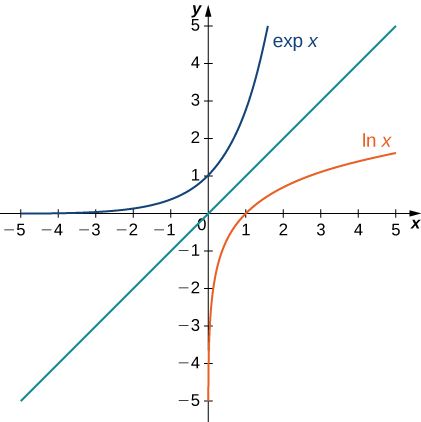## Exponential and Logarithmic Functions

### Learning Outcomes

• Recognize the derivative and integral of the exponential function.
• Prove properties of logarithms and exponential functions using integrals.
• Express general logarithmic and exponential functions in terms of natural logarithms and exponentials.

## The Exponential Function

We now turn our attention to the function ${e}^{x}.$ Note that the natural logarithm is one-to-one and therefore has an inverse function. For now, we denote this inverse function by $\text{exp}x.$ Then,

$\text{exp}(\text{ln}x)=x\text{ for }x>0\text{ and }\text{ln}(\text{exp}x)=x\text{ for all }x.$

The following figure shows the graphs of $\text{exp}x$ and $\text{ln}x.$Figure 4. The graphs of $\text{ln}x$ and $\text{exp}x.$

We hypothesize that $\text{exp}x={e}^{x}.$ For rational values of $x,$ this is easy to show. If $x$ is rational, then we have $\text{ln}({e}^{x})=x\text{ln}e=x.$ Thus, when $x$ is rational, ${e}^{x}=\text{exp}x.$ For irrational values of $x,$ we simply define ${e}^{x}$ as the inverse function of $\text{ln}x.$

### Definition

For any real number $x,$ define $y={e}^{x}$ to be the number for which

$\text{ln}y=\text{ln}({e}^{x})=x$

Then we have ${e}^{x}=\text{exp}(x)$ for all $x,$ and thus

${e}^{\text{ln}x}=x\text{ for }x>0\text{ and }\text{ln}({e}^{x})=x$

for all $x.$

## Properties of the Exponential Function

Since the exponential function was defined in terms of an inverse function, and not in terms of a power of $e,$ we must verify that the usual laws of exponents hold for the function ${e}^{x}.$

### Properties of the Exponential Function

If $p$ and $q$ are any real numbers and $r$ is a rational number, then

1. ${e}^{p}{e}^{q}={e}^{p+q}$
2. $\frac{{e}^{p}}{{e}^{q}}={e}^{p-q}$
3. ${({e}^{p})}^{r}={e}^{pr}$

### Proof

Note that if $p$ and $q$ are rational, the properties hold. However, if $p$ or $q$ are irrational, we must apply the inverse function definition of ${e}^{x}$ and verify the properties. Only the first property is verified here; the other two are left to you. We have

$\text{ln}({e}^{p}{e}^{q})=\text{ln}({e}^{p})+\text{ln}({e}^{q})=p+q=\text{ln}({e}^{p+q}).$

Since $\text{ln}x$ is one-to-one, then

${e}^{p}{e}^{q}={e}^{p+q}.$

$_\blacksquare$

As with part iv. of the logarithm properties, we can extend property iii. to irrational values of $r,$ and we do so by the end of the section.

We also want to verify the differentiation formula for the function $y={e}^{x}.$ To do this, we need to use implicit differentiation. Let $y={e}^{x}.$ Then

$\begin{array}{ccc}\hfill \text{ln}y& =\hfill & x\hfill \\ \hfill \frac{d}{dx}\text{ln}y& =\hfill & \frac{d}{dx}x\hfill \\ \hfill \frac{1}{y}\frac{dy}{dx}& =\hfill & 1\hfill \\ \hfill \frac{dy}{dx}& =\hfill & y.\hfill \end{array}$

Thus, we see

$\frac{d}{dx}{e}^{x}={e}^{x}$

as desired, which leads immediately to the integration formula

$\displaystyle\int {e}^{x}dx={e}^{x}+C$

We apply these formulas in the following examples.

### Example: Using Properties of Exponential Functions

Evaluate the following derivatives:

1. $\frac{d}{dt}{e}^{3t}{e}^{{t}^{2}}$
2. $\frac{d}{dx}{e}^{3{x}^{2}}$

### Try It

Evaluate the following derivatives:

1. $\frac{d}{dx}(\frac{{e}^{{x}^{2}}}{{e}^{5x}})$
2. $\frac{d}{dt}{({e}^{2t})}^{3}$

Watch the following video to see the worked solution to the above Try It.

### Example: Using Properties of Exponential Functions

Evaluate the following integral: $\displaystyle\int 2x{e}^{\text{−}{x}^{2}}dx.$

### Try It

Evaluate the following integral: $\displaystyle\int \frac{4}{{e}^{3x}}dx.$

Watch the following video to see the worked solution to the above Try It.

## General Logarithmic and Exponential Functions

We close this section by looking at exponential functions and logarithms with bases other than $e.$ Exponential functions are functions of the form $f(x)={a}^{x}.$ Note that unless $a=e,$ we still do not have a mathematically rigorous definition of these functions for irrational exponents. Let’s rectify that here by defining the function $f(x)={a}^{x}$ in terms of the exponential function ${e}^{x}.$ We then examine logarithms with bases other than $e$ as inverse functions of exponential functions.

### Definition

For any $a>0,$ and for any real number $x,$ define $y={a}^{x}$ as follows:

$y={a}^{x}={e}^{x\text{ln}a}$

Now ${a}^{x}$ is defined rigorously for all values of $x$. This definition also allows us to generalize property iv. of logarithms and property iii. of exponential functions to apply to both rational and irrational values of $r.$ It is straightforward to show that properties of exponents hold for general exponential functions defined in this way.

Let’s now apply this definition to calculate a differentiation formula for ${a}^{x}.$ We have

$\frac{d}{dx}{a}^{x}=\frac{d}{dx}{e}^{x\text{ln}a}={e}^{x\text{ln}a}\text{ln}a={a}^{x}\text{ln}a.$

The corresponding integration formula follows immediately.

### Derivatives and Integrals Involving General Exponential Functions

Let $a>0.$ Then,

$\frac{d}{dx}{a}^{x}={a}^{x}\text{ln}a$

and

$\displaystyle\int {a}^{x}dx=\frac{1}{\text{ln}a}{a}^{x}+C$

If $a\ne 1,$ then the function ${a}^{x}$ is one-to-one and has a well-defined inverse. Its inverse is denoted by ${\text{log}}_{a}x.$ Then,

$y={\text{log}}_{a}x\text{if and only if}x={a}^{y}$

Note that general logarithm functions can be written in terms of the natural logarithm. Let $y={\text{log}}_{a}x.$ Then, $x={a}^{y}.$ Taking the natural logarithm of both sides of this second equation, we get

$\begin{array}{ccc}\hfill \text{ln}x& =\hfill & \text{ln}({a}^{y})\hfill \\ \hfill \text{ln}x& =\hfill & y\text{ln}a\hfill \\ \hfill y& =\hfill & \frac{\text{ln}x}{\text{ln}a}\hfill \\ \hfill {\text{log}}_{}x& =\hfill & \frac{\text{ln}x}{\text{ln}a}.\hfill \end{array}$

Thus, we see that all logarithmic functions are constant multiples of one another. Next, we use this formula to find a differentiation formula for a logarithm with base $a.$ Again, let $y={\text{log}}_{a}x.$ Then,

$\begin{array}{cc}\hfill \frac{dy}{dx}& =\frac{d}{dx}({\text{log}}_{a}x)\hfill \\ & =\frac{d}{dx}(\frac{\text{ln}x}{\text{ln}a})\hfill \\ & =(\frac{1}{\text{ln}a})\frac{d}{dx}(\text{ln}x)\hfill \\ & =\frac{1}{\text{ln}a}·\frac{1}{x}\hfill \\ & =\frac{1}{x\text{ln}a}.\hfill \end{array}$

### Derivatives of General Logarithm Functions

Let $a>0.$ Then,

$\frac{d}{dx}{\text{log}}_{a}x=\frac{1}{x\text{ln}a}$

### Example: Calculating Derivatives of General Exponential and Logarithm Functions

Evaluate the following derivatives:

1. $\frac{d}{dt}({4}^{t}·{2}^{{t}^{2}})$
2. $\frac{d}{dx}{\text{log}}_{8}(7{x}^{2}+4)$

### Try It

Evaluate the following derivatives:

1. $\frac{d}{dt}{4}^{{t}^{4}}$
2. $\frac{d}{dx}{\text{log}}_{3}(\sqrt{{x}^{2}+1})$

Watch the following video to see the worked solution to the above Try It.

### Example: Integrating General Exponential Functions

Evaluate the following integral: $\displaystyle\int \frac{3}{{2}^{3x}}dx.$

### Try It

Evaluate the following integral: $\displaystyle\int {x}^{2}{2}^{{x}^{3}}dx.$

Watch the following video to see the worked solution to the above Try It.